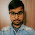### Program 223:Decryption of Rail Fence Cipher using C

Program 223:

```#include<stdio.h>
#include<string.h>
#include<stdlib.h>
main()
{
int i,j,k,len,rails,count,code;
char str;
printf("Enter a Secret Message\n");
gets(str);

len=strlen(str);

printf("Enter number of rails\n");
scanf("%d",&rails);
for(i=0;i<rails;i++)
{
for(j=0;j<len;j++)
{
code[i][j]=0;
}
}
count=0;
j=0;
while(j<len)
{
if(count%2==0)
{
for(i=0;i<rails;i++)
{
//strcpy(code[i][j],str[j]);
code[i][j]=-1;
j++;
}

}
else
{

for(i=rails-2;i>0;i--)
{
code[i][j]=-1;
j++;
}
}

count++;
}
//Decrypting here.Above is same as encryption but instead of keeping letters we stored it with -1 i.e. dashes(in view)
k=0;
for(i=0;i<rails;i++)
{
for(j=0;j<len;j++)
{
if(code[i][j]!=0)
{
code[i][j]=(int)str[k];
k++;
}
}
}
//repeating above procedure
count=0;
j=0;
while(j<len)
{
if(count%2==0)
{
for(i=0;i<rails;i++)
{
//strcpy(code[i][j],str[j]);
printf("%c",code[i][j]);
j++;
}

}
else
{

for(i=rails-2;i>0;i--)
{
printf("%c",code[i][j]);
j++;
}
}

count++;
}
printf("\n");
}

```
Explanation:
Pre explanation of logic:-

//Coming Soon

Output: Should consider even Spaces

1.thanks, it works

2.This comment has been removed by the author.

3.Wall posts can be driven in with a demo hammer and may have pre-made snares on them to draw in the openings in the fencing.
Fence repair

4.Your cherished pets can likewise be safeguarded by the pool fencing.
Aurora Fence Pros

 Donate Buy me a coffee \$2.00 USD Buy me a burger \$5.00 USD Buy me a pizza \$10.00 USDCompiler Used by me C-Free 5.0(Recommended) Other Compilers Code Blocks(Recommended) Online Compilers Ideone(Remember to give input before executing online where ever necessary)